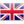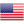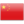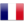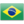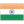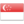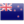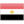» » » Hoesch Z-type sheet pile

Hoesch Z-type sheet pile

Views:10

Z-type sheet pile is usually used as anchorage structure, and the strength calculation of sheet pile wall is the same as that of sheet pile wall. In other words, in the case of hot rolled Z-shaped sheet pile manufacturers, the value obtained by multiplying the calculated standard load value by the comprehensive partial load factor shall be less than or equal to the design value specified in this Code. For reinforced concrete members, the comprehensive partial load factor of strength check calculation should be 1. Crack calculation and crack width calculation, and the comprehensive partial load factor should be 0.85.

Anchor walls usually adopt continuous structures. The Z-type sheet pile tension RA is used as the support response, and the method of multi-span rigidly supported continuous beam is used to calculate. The continuous beam can be arranged in the middle of the wall (vertical). The Rer A here should be obtained by multiplying the load of the standard value by the non-uniformity coefficient of the pull force of the tie rod = 1.35, that is, RA = RA = 1.35 RA. If the anchor plate is continuous, the calculation results are in accordance with the hot rolled Z-shaped sheet pile. If multiple tie rods share a plate, the calculation of the beam should be a continuous beam with multi-span rigid support, and the plate surface should be calculated according to the cantilever plates at both ends. When a tie rod corresponds to a plate, if there is a suspension beam, the calculation of the main beam and the plate should be cantilever plate; if there is no beam, the punching calculation should be checked outside the plate, and the attachment of the tie rod hole should be equipped with reinforcing bars.

Horizontal displacement of anchored walls or slabs can be implemented in accordance with relevant codes. The length and internal force of anchored single sheet pile can be calculated by using the calculation method of sheet-pile wall without anchor plate under concentrated horizontal force Ra. Hoesch Z sheet pile is produced by ThyssenKrupp.

Contact with us

Shunli Steel Group
Tel. : 0086 -25 - 84722733
Fax: 0086 - 25 - 84730966
Hand phone: +86 13905190622
001 9023936528（Canada & USA）

QQ: 477798703